Updating search results...

# 27 Results

View
Selected filters:
• Equation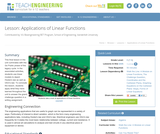Read the Fine Print
Educational Use
Rating
0.0 stars

This final lesson in the unit culminates with the Go Public phase of the legacy cycle. In the associated activities, students use linear models to depict Hooke's law as well as Ohm's law. To conclude the lesson, students apply they have learned throughout the unit to answer the grand challenge question in a writing assignment.

Subject:
Engineering
Mathematics
Algebra
Material Type:
Lesson Plan
Provider:
TeachEngineering
Provider Set:
TeachEngineering
Author:
Aubrey McKelvey
Date Added:
09/18/2014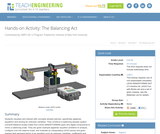Read the Fine Print
Educational Use
Rating
0.0 stars

Students visualize and interact with concepts already learned, specifically algebraic equations and solving for unknown variables. They construct a balancing seesaw system (LEGO® Balance Scale) made from LEGO MINDSTORMS® parts and digital components to mimic a balancing scale. They are given example algebraic equation problems to analyze, configure onto the balance scale, and evaluate by manipulating LEGO pieces and gram masses that represent terms of an equation such as unknown variables, coefficients and integers. Digital light sensors, built into the LEGO Balance Scale, detect any balance or imbalances displayed on the balancing scale. The LEGO Balance Scale interactively issues a digital indication of balance or imbalance within the system. If unbalanced, students continue using the LEGO Balance Scale until they are confident in their understanding of solving algebraic equations. The goal is for students to become confident in solving algebraic equations by fundamentally understanding the basics of algebra and real-world algebraic applications.

Subject:
Engineering
Chemistry
Material Type:
Activity/Lab
Provider:
TeachEngineering
Provider Set:
TeachEngineering
Author:
Gisselle Cunningham
Jared Soto
Linderick Outerbridge
Russell Holstein
Timothy Li
Date Added:
09/18/2014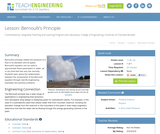Read the Fine Print
Educational Use
Rating
0.0 stars

Bernoulli's principle relates the pressure of a fluid to its elevation and its speed. Bernoulli's equation can be used to approximate these parameters in water, air or any fluid that has very low viscosity. Students learn about the relationships between the components of the Bernoulli equation through real-life engineering examples and practice problems.

Subject:
Engineering
Physics
Material Type:
Lesson Plan
Provider:
TeachEngineering
Provider Set:
TeachEngineering
Author:
Denise W. Carlson
James Prager
Karen King
Date Added:
09/18/2014Unrestricted Use
CC BY
Rating
0.0 stars

A teacher's guide to the connection between the definition and equation of an ellipse, and how to get from one to the other.

Subject:
Algebra
Material Type:
Teaching/Learning Strategy
Provider:
Rice University
Provider Set:
Connexions
Author:
Kenny Felder
Date Added:
02/16/2011Unrestricted Use
CC BY
Rating
0.0 stars

A teacher's guide on teaching the connection between the definition and equation of a parabola, and how to get from one to the other.

Subject:
Algebra
Material Type:
Teaching/Learning Strategy
Provider:
Rice University
Provider Set:
Connexions
Author:
Kenny Felder
Date Added:
02/16/2011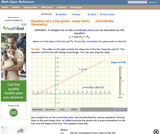Read the Fine Print
Rating
0.0 stars

An interactive applet and associated web page that demonstrate the equation of a line in point-slope form. The user can move a slider that controls the slope, and can drag the point that defines the line. The graph changes accordingly and equation for the line is continuously recalculated with every slider and / or point move. The grid, axis pointers and coordinates can be turned on and off. The equation display can be turned off to permit class exercises and then turned back on the verify the answers. The applet can be printed as it appears on the screen to make handouts. The web page has a full description of the concept of the equation of a line in point - slope form, a worked example and has links to other pages relating to coordinate geometry. Applet can be enlarged to full screen size for use with a classroom projector. This resource is a component of the Math Open Reference Interactive Geometry textbook project at http://www.mathopenref.com.

Subject:
Geometry
Material Type:
Reading
Simulation
Provider:
Math Open Reference
Author:
John Page
Date Added:
02/16/2011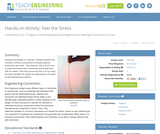Read the Fine Print
Educational Use
Rating
0.0 stars

Working individually or in groups, students explore the concept of stress (compression) through physical experience and math. They discover why it hurts more to poke themselves with mechanical pencil lead than with an eraser. Then they prove why this is so by using the basic equation for stress and applying the concepts to real engineering problems.

Subject:
Engineering
Life Science
Mathematics
Geometry
Physics
Material Type:
Activity/Lab
Provider:
TeachEngineering
Provider Set:
TeachEngineering
Author:
Jeffrey Mitchell
Date Added:
09/18/2014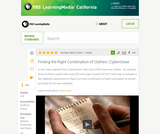Read the Fine Print
Educational Use
Rating
0.0 stars

In this video segment from Cyberchase, Harry has a fixed budget for clothing, so he must figure out what combination of jackets and pants he can buy with \$100.

Subject:
Mathematics
Algebra
Material Type:
Lecture
Provider:
PBS LearningMedia
Provider Set:
PBS Learning Media: Multimedia Resources for the Classroom and Professional Development
Author:
U.S. Department of Education
WNET
Date Added:
07/08/2008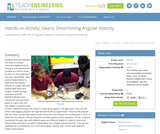Read the Fine Print
Educational Use
Rating
0.0 stars

Students work as engineers and learn to conduct controlled experiments by changing one experimental variable at a time to study its effect on the experiment outcome. Specifically, they conduct experiments to determine the angular velocity for a gear train with varying gear ratios and lengths. Student groups assemble LEGO MINDSTORMS(TM) NXT robots with variously sized gears in a gear train and then design programs using the NXT software to cause the motor to rotate all the gears in the gear train. They use the LEGO data logging program and light sensors to set up experiments. They run the program with the motor and the light sensor at the same time and analyze the resulting plot in order to determine the angular velocity using the provided physics-based equations. Finally, students manipulate the gear train with different gears and different lengths in order to analyze all these factors and figure out which manipulation has a higher angular velocity. They use the equations for circumference of a circle and angular velocity; and convert units between radians and degrees.

Subject:
Engineering
Material Type:
Activity/Lab
Provider:
TeachEngineering
Provider Set:
TeachEngineering
Author:
James Cox
Jasmin Mejias
Jennifer S. Haghpanah
Leonarda Huertas
Mihai Pruna
Date Added:
09/18/2014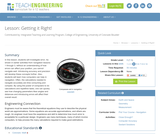Read the Fine Print
Educational Use
Rating
0.0 stars

In this lesson, students will investigate error. As shown in earlier activities from navigation lessons 1 through 3, without an understanding of how errors can affect your position, you cannot navigate well. Introducing accuracy and precision will develop these concepts further. Also, students will learn how computers can help in navigation. Often, the calculations needed to navigate accurately are time consuming and complex. By using the power of computers to do calculations and repetitive tasks, one can quickly see how changing parameters likes angles and distances and introducing errors will affect their overall result.

Subject:
Engineering
Mathematics
Geometry
Trigonometry
Material Type:
Activity/Lab
Lesson Plan
Provider:
TeachEngineering
Provider Set:
TeachEngineering
Author:
Janet Yowell
Jeff White
Malinda Schaefer Zarske
Matt Lippis
Penny Axelrad
Date Added:
09/18/2014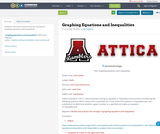Conditional Remix & Share Permitted
CC BY-NC
Rating
0.0 stars

Students will review and practice the concepts of graphing equations and inequalities

Subject:
Mathematics
Material Type:
Activity/Lab
Author:
Joe Ferguson
Date Added:
07/10/2017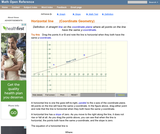Read the Fine Print
Rating
0.0 stars

An interactive applet and associated web page that show the definition of a horizontal line in coordinate geometry. The applet has two points that the user can drag which define a line. The line flagged when it is horizontal (slope=0) and the equation of the line is shown. The grid, details and coordinates can be turned on and off. The applet can be printed exactly as it appears on the screen to make handouts. The web page has a discussion on how to test for horizontal, the line equation and has links to other pages relating to coordinate geometry. Applet can be enlarged to full screen size for use with a classroom projector. This resource is a component of the Math Open Reference Interactive Geometry textbook project at http://www.mathopenref.com.

Subject:
Geometry
Material Type:
Reading
Simulation
Provider:
Math Open Reference
Author:
John Page
Date Added:
02/16/2011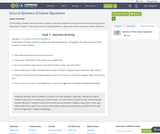Conditional Remix & Share Permitted
CC BY-NC
Rating
0.0 stars

In this activity, students write and solve a system of two linear equations to explore the numerical and graphical meaning of "solution." The activity closes by asking students to apply what they've learned to similar situations.

Subject:
Mathematics
Algebra
Material Type:
Lesson Plan
Author:
Shawn Dykstra
Date Added:
11/14/2017
Remix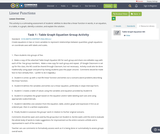Conditional Remix & Share Permitted
CC BY-NC
Rating
0.0 stars

This activity is a culmnating assessment of students' abilities to describe a linear function in words, in an equation, in a table, in a graph, identify a solution, and explain the solution.

Subject:
Educational Technology
Mathematics
Algebra
Material Type:
Lesson Plan
Author:
Shawn Dykstra
Date Added:
11/14/2017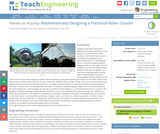Read the Fine Print
Educational Use
Rating
0.0 stars

Students apply high school-level differential calculus and physics to the design of two-dimensional roller coasters in which the friction force is considered, as explained in the associated lesson. In a challenge the mirrors real-world engineering, the designed roller coaster paths must be made from at least five differentiable functions that are put together such that the resulting piecewise curving path is differentiable at all points. Once designed mathematically, teams build and test small-sized prototype models of the exact designs using foam pipe wrap insulation as the roller coaster track channel with marbles as the ride carts.

Subject:
Engineering
Algebra
Measurement and Data
Physics
Material Type:
Activity/Lab
Provider:
TeachEngineering
Provider Set:
Activities
Author:
Miguel R. Ramirez
Date Added:
08/31/2017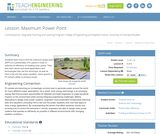Read the Fine Print
Educational Use
Rating
0.0 stars

Students learn how to find the maximum power point (MPP) of a photovoltaic (PV) panel in order to optimize its efficiency at creating solar power. They also learn about real-world applications and technologies that use this technique, as well as Ohm's law and the power equation, which govern a PV panel's ability to produce power.

Subject:
Engineering
Environmental Science
Material Type:
Lesson Plan
Provider:
TeachEngineering
Provider Set:
TeachEngineering
Author:
Abby Watrous
Jack Baum
Malinda Schaefer Zarske
Stephen Johnson
William Surles
Date Added:
09/18/2014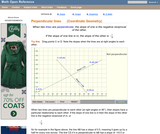Read the Fine Print
Rating
0.0 stars

An interactive applet and associated web page that show how to determine of one line is perpendicular to another in coordinate geometry. The principle used is that if two lines a re perpendicular to each other the slope of one is the negative reciprocal of the other. The applet shows to lines that the user can move. The slopes are continuously calculated as you drag them, and if the they are parallel they change color. The calculation is shown on screen updated continuously as you drag. The grid, axis pointers and coordinates can be turned on and off. The calculation display can be turned off to permit class exercises and then turned back on the verify the answers. The applet can be printed as it appears on the screen to make handouts. The web page has a full description of the concept of perpendicularity, a worked example and has links to other pages relating to coordinate geometry. Applet can be enlarged to full screen size for use with a classroom projector. This resource is a component of the Math Open Reference Interactive Geometry textbook project at http://www.mathopenref.com.

Subject:
Geometry
Material Type:
Reading
Simulation
Provider:
Math Open Reference
Author:
John Page
Date Added:
02/16/2011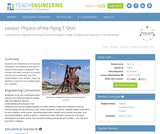Read the Fine Print
Educational Use
Rating
0.0 stars

Students are introduced to the physics concepts of air resistance and launch angle as they apply to catapults. This includes the basic concepts of position, velocity and acceleration and their relationships to one another. They use algebra to solve for one variable given two variables.

Subject:
Engineering
Physics
Material Type:
Lesson Plan
Provider:
TeachEngineering
Provider Set:
TeachEngineering
Author:
Brandi Jackson
Denise W. Carlson
Jonathan MacNeil
Scott Duckworth
Stephanie Rivale
Date Added:
09/18/2014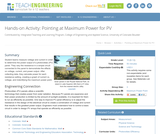Read the Fine Print
Educational Use
Rating
0.0 stars

Student teams measure voltage and current in order to determine the power output of a photovoltaic (PV) panel. They vary the resistance in a simple circuit connected to the panel to demonstrate the effects on voltage, current, and power output. After collecting data, they calculate power for each resistance setting, creating a graph of current vs. voltage, and indentifying the maximum power point.

Subject:
Engineering
Electronic Technology
Material Type:
Activity/Lab
Provider:
TeachEngineering
Provider Set:
TeachEngineering
Author:
Abby Watrous
Eszter Horanyi
Jack Baum
Malinda Schaefer Zarske
Stephen Johnson
William Surles
Date Added:
09/18/2014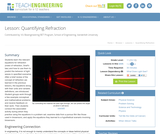Read the Fine Print
Educational Use
Rating
0.0 stars

Students learn the relevant equations for refraction (index of refraction, Snell's law) and how to use them to predict the behavior of light waves in specified scenarios. After a brief review of the concept of refraction (as learned in the previous lesson), the equations along with their units and variable definitions, are introduced. Student groups work through a few example conceptual and mathematical problems and receive feedback on their work. Then students conduct the associated activity during which they practice using the equations in a problem set, examine data from a porous film like those used in biosensors, and apply the equations they learned to a hypothetical scenario involving biosensors.

Subject:
Engineering
Physics
Material Type:
Lesson Plan
Provider:
TeachEngineering
Provider Set:
TeachEngineering
Author:
Caleb Swartz
Date Added:
09/18/2014